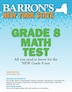# Barron's New York State Grade 8 Math Test

## byAnne M. Szczesny

### Pricing and Purchase Info

\$15.99

Earn 80 plum® points

Prices and offers may vary in store

Quantity:

In stock online

Ships free on orders over \$25

Not available in stores

This revised and updated test preparation manual reviews all Grade 8 math topics covered on the new New York State Math Assessment test. They include the number system; measurement; fractions, decimals, and percents; the basics of geometry and trigonometry; transformations; equations; and graphing. This book also presents test-taking advice and two sample tests with solutions to all problems. Helpful diagrams and line illustrations appear throughout the book.
Title:Barron's New York State Grade 8 Math TestFormat:PaperbackDimensions:240 pages, 10 × 7.75 × 0.5 inPublished:August 1, 2011Publisher:Barrons Educational SeriesLanguage:English

The following ISBNs are associated with this title:

ISBN - 10:0764146238

ISBN - 13:9780764146237

Look for similar items by category:

BooksReference and LanguageStudy Aids

## Reviews

• CHAPTER 1 THE TEST

Scoring

Test-Taking Tips
Sample Questions

• CHAPTER 2 NUMBERS AND OPERATIONS

Number Sets

Properties of Operations

Absolute Value

Operations on Integers

Order of Operations

Adding and Subtracting Fractions

Multiplying and Dividing Fractions

Divisibility Rules

Greatest Common Factor and Least Common Multiple

Prime Numbers, Composite Numbers, and Exponents

Scientific Notation

• CHAPTER 3 PATTERNS AND FUNCTIONS

Writing Expressions

Evaluating Expressions

Writing Expressions and Equations from Data

Equations and Inequalities

Solving Equations

Solving and Graphing Inequalities

Writing Equations and Inequalities from Word Problems

• CHAPTER 4 RATIO, PROPORTION, AND PERCENT

Ratio and Proportion

Changing Fractions to Decimals

Changing Decimals to Percents

Changing Fractions to Percents

Solving Percent Problems

Interest

• CHAPTER 5 MEASUREMENT

Customary System

Metric System

Converting Between Systems

Temperature Conversion

Monetary Conversion

• CHAPTER 6 MONOMIALS AND POLYNOMIALS

Subtracting Polynomials

Laws of Exponents

Multiplying a Monomial by a Monomial

Multiplying a Binomial by a Monomial

Squaring a Monomial

Multiplying a Binomial by a Binomial

Squaring a Binomial

Dividing a Polynomial by a Monomial

Factoring Algebraic Expressions

Factoring Trinomials of the Form ax2 + bx + c

• CHAPTER 7 GEOMETRY

Lines

Angles

Plane Figures

Right Triangles

Other Polygons

Interior Angles of Polygons

Similarity and Congruence

• CHAPTER 8 GRAPHING

The Coordinate System

Plotting Points

Slope

Graphing Lines

Writing Equations of Lines

Solving Systems of Equations

Graphing Linear Inequalities

Relations and Functions

Descriptive Graphs

• CHAPTER 9 TRANSFORMATIONS

Translation

Symmetry

Reflection

Rotation

Dilation

• CHAPTER 10 SOLUTIONS TO PRACTICE PROBLEMS

• CHAPTER 11 SAMPLE TEST 1

Answers to Sample Test 1

• CHAPTER 12 SAMPLE TEST 2

Answers to Sample Test 2

Index